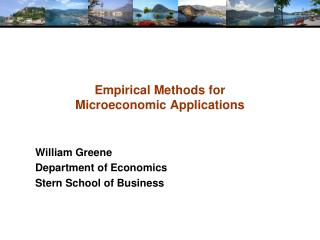DownloadDownload PresentationEmpirical Methods for Microeconomic Applications

# Empirical Methods for Microeconomic Applications

Télécharger la présentation## Empirical Methods for Microeconomic Applications

- - - - - - - - - - - - - - - - - - - - - - - - - - - E N D - - - - - - - - - - - - - - - - - - - - - - - - - - -
##### Presentation Transcript

1. Empirical Methods for Microeconomic Applications William Greene Department of Economics Stern School of Business

2. Lab 4. Ordered Choice and Count Data

4. Binary Dependent Variables DOCTOR = visited the doctor at least once HOSPITAL = went to the hospital at least once. PUBLIC = has public health insurance (1=YES) ADDON = additional health insurance.(1=Yes) ADDON is extremely unbalanced.

5. Ordered Dependent Variable HSAT = ordered reported health satisfaction, coded 0,1,…,10. Use with ORDERED or ORDERED ; Logit or OPROBIT and OLOGIT

6. HISTOGRAM ; Rhs = hsat \$

7. HISTOGRAM ; Rhs = hsat ; Group = female ; Labels = Male,Female ; Title = Health Satisfaction by Gender \$

8. Ordered Choice Models Ordered ; Lhs = dependent variable ; Rhs = One, … independent variables \$ Remember to include the constant term For ordered logit i stead of ordered probit, use Ordered ; Logit ; Lhs = dependent variable ; Rhs = One, … independent variables \$ To get marginal effects, use ; Margin as usual. There are fixed and random effects estimators for this model: ; FEM ; Panel ; Random ; Panel

9. Sample Selection in Ordered Choice

10. Sample Selection Ordered Probit PROBIT ; Lhs = … ; Rhs = … ; HOLD \$ ORDERED ; Lhs = … ; Rhs = … ; Selection \$ This is a maximum likelihood estimator, not a least squares estimator. There is no ‘lambda’ variable. The various parameters are present in the likelihood function.

11. PROBIT;if[year1994=1]; lhs=public;rhs=one,age,income,working ;hold\$ORDERED; If[year1994=1] ; lhs=health;rhs=x;selection\$

12. Hierarchical Ordered Probit Hierarchical ordered probit. Ordered probit in which threshold parameters depend on variables. Two forms: HO1: μ(i,j) = exp[θ(j) + δ’z(i)]. HO2, different δ vector for each j. Use ORDERED ; … ; HO1 = list of variables or ORDERED ; … ; HO2 = list of variables. Can combine with SELECTION models and ZERO INFLATION models. This is also the Pudney and Shields generalized ordered probit from Journal of Applied Econometrics, August 2000, with the modification of using exp(…) and internally, a way to make sure that the thresholds are ordered..

13. Count Data DOCVIS = count of visits to the doctor HOSPVIS = count of visits to the hospital. There are outliers. It helps to use truncated or censored samples (1) Truncated Data SAMPLE ; All \$ MODEL ; If [ docvis <= 10] ; Lhs = … \$ (2) Censored Data SAMPLE ; All \$ CREATE ; DocVis10 = Min(10,DocVis) ; Hosp10 = Min(10,HospVis) \$ There are also excess zeros. ZIP or HURDLE model suggested.

14. Models for Count Data • Basic models Poisson and negative binomial POISSON ; Lhs = y ; Rhs = One,… \$ NEGBIN ; Lhs = y ; Rhs = One,… \$ • NEGBIN ; Model=NB1 or NB2 or NBP ; … • Many extensions • Various heterogeneity forms • Panel data • Random parameters and latent class • Zero inflation • Sample selection • Censoring and truncation • Numerous others… (some, far from all, shown below)

15. NEGBIN ; If[year1994=1];Lhs=docvis;rhs=x;model=nbp\$

16. Sample Selection

17. Selection Model Probit ; Lhs = … ; Rhs = … ; Hold \$ Poisson ; Lhs = … ; Rhs = … ; Selection ; MLE\$

18. Zero Inflation Two regime, latent class model • Prob[Regime 1 => y=0] = q • Prob[y = j|Regime 2] = Poisson or NegBin, λ=exp(β’x) Reduced form: • Prob[y=0] = q + (1-q)P(0) • Prob[y=j > 0] = (1-q)P(j) Regime Models: • q = Probit or Logit • Structures: • ZIP: Probit or Logit F(γ’z) z can be any set of variables • ZIP-tau: Probit or Logit F(τ β’x) – same β’x as above

19. ZIP and ZIP-tau Models ;ZIP Logit, ZIP-tau ;ZIP = Normal: Probit ZIP-tau ;ZIP [=Normal] ; Rh2 = variables in z Alternative Models • Default is Poisson • ; MODEL = NegBin • ; MODEL = Gamma

20. Hurdle Model Two Part Model: • Prob[y=0] = Logit or Probit using β’x from the count model or γ’z as specified with ;RH2=list • Prob[y=j|j>0] = Truncated Poisson or NegBin Two part decision: POISSON ; … ; Hurdle \$ POISSON ; … ; Hurdle ; Rh2 = List \$

21. POISSON ; If[year1994=1]; Lhs = docvis;rhs=x ; hurdle ; Rh2 = one,age,income,working \$PARTIALS ; If[year1994=1] ; effects: x ; summary \$

22. Poisson and NB Mixture Models with Normal Heterogeneity